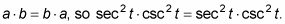##### Trigonometry Workbook For DummiesWhen the terms in a trig proof are being added or subtracted, you may create fractions where none were before. This is especially true when dealing with secant and cosecant, because you create fractions when you convert them (respectively) to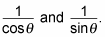The same is true for tangent when you change it to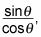and cotangent becomes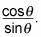Here’s an example that illustrates this point. Follow these steps to prove that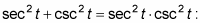1. Convert all the trig functions to sines and cosines.

On the left side, you now have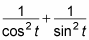2. Find the lowest common denominator of the two fractions.

This multiplication gives you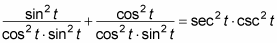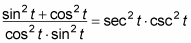4. Simplify the expression with a Pythagorean identity in the numerator.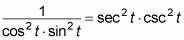5. Use reciprocal identities to invert the fraction.

Both sides now have multiplication: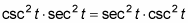Keep in mind that some pre-calculus teachers let you stop there; others, however, make you rewrite the equation so that the left and right sides match exactly. Every teacher has his or her own way of proving trig identities. Make sure that you adhere to your teacher’s expectations; otherwise, you may lose points on a test.

6. Use the properties of equality to rewrite.

The commutative property of multiplication says that